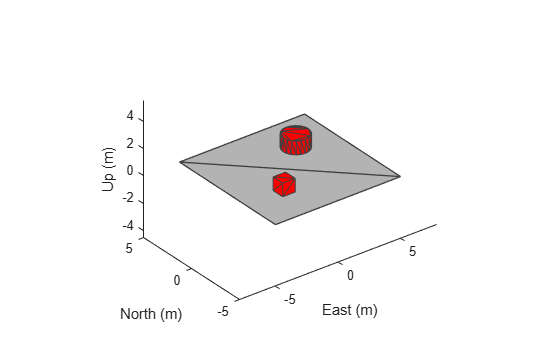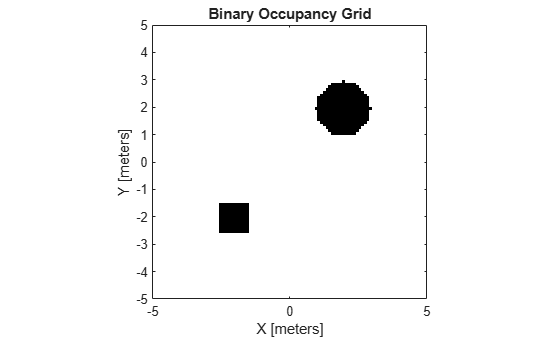# binaryOccupancyMap

Create 2-D binary occupancy map from robot scenario

## Syntax

``map = binaryOccupancyMap(scenario,Name=Value)``

## Description

example

````map = binaryOccupancyMap(scenario,Name=Value)` creates binary occupancy map based on mesh elements from scenario defined with `IsBinaryOccupied` status `true`. The mesh elements are processed in the 3-D convex hull form. Further, mesh element is considered as occupied region only if it lies inside map height limits and map size. These properties are specified by one or more name-value pair arguments.```

## Examples

collapse all

Create a robot scenario.

`scenario = robotScenario(UpdateRate=1,StopTime=10);`

Add a plane, box and cylinder mesh in the scenario.

```addMesh(scenario,"Plane",Size=[10 10],Color=[0.7 0.7 0.7]) addMesh(scenario,"Box",Position=[-2 -2 0.5],IsBinaryOccupied=true) addMesh(scenario,"Cylinder",Position=[2 2 0.5],IsBinaryOccupied=true)```

Visualize the scenario.

`show3D(scenario);`Get the 2-D occupancy map.

```occupancyMap = binaryOccupancyMap(scenario,MapHeightLimits=[-1 1], ... GridOriginInLocal=[-5 -5]);```

Visualize the 2-D occupancy map.

```figure show(occupancyMap);```## Input Arguments

collapse all

Robot scenario, specified as a `robotScenario` object.

### Name-Value Arguments

Specify optional pairs of arguments as `Name1=Value1,...,NameN=ValueN`, where `Name` is the argument name and `Value` is the corresponding value. Name-value arguments must appear after other arguments, but the order of the pairs does not matter.

Before R2021a, use commas to separate each name and value, and enclose `Name` in quotes.

Example: ```map = binaryOccupancyMap(scenario,MapResolution=15)```

Origin of occupancy map grid in local coordinates, specified as a two-element vector of the form ```[xLocal yLocal]``` in meters.

Data Types: `single` | `double`

Resolution of occupancy map grid in z-axis, specified as a scalar integer in cells per meter.

Data Types: `single` | `double`

Minimum and maximum values of map height, specified as a two-element vector of the form ```[Hmin Hmax]``` in meters, from which static meshes are considered for occupancy map grid.

Data Types: `single` | `double`

Resolution of occupancy map grid in xy-axis, specified as a scalar integer in cells per meter.

Data Types: `single` | `double`

Size of occupancy map grid, specified as a two-element vector of the form ```[width height]``` in meters.

Data Types: `single` | `double`

## Output Arguments

collapse all

Binary occupancy map from robot scenario, returned as a `binaryOccupancyMap` object.

## Version History

Introduced in R2022a